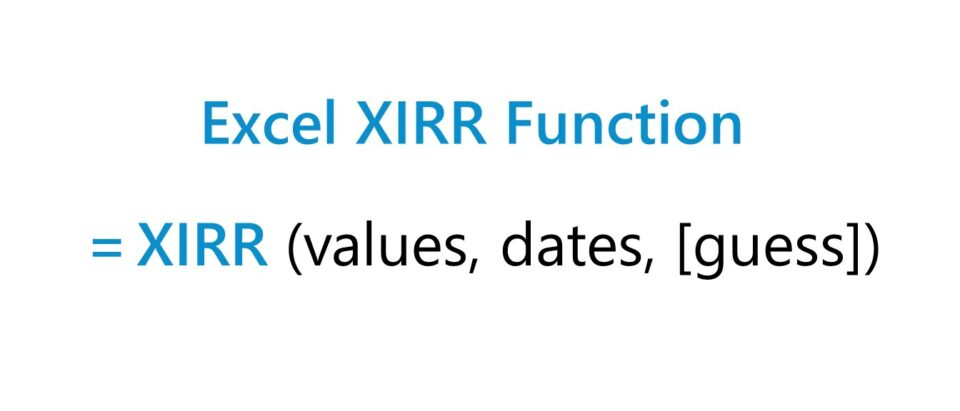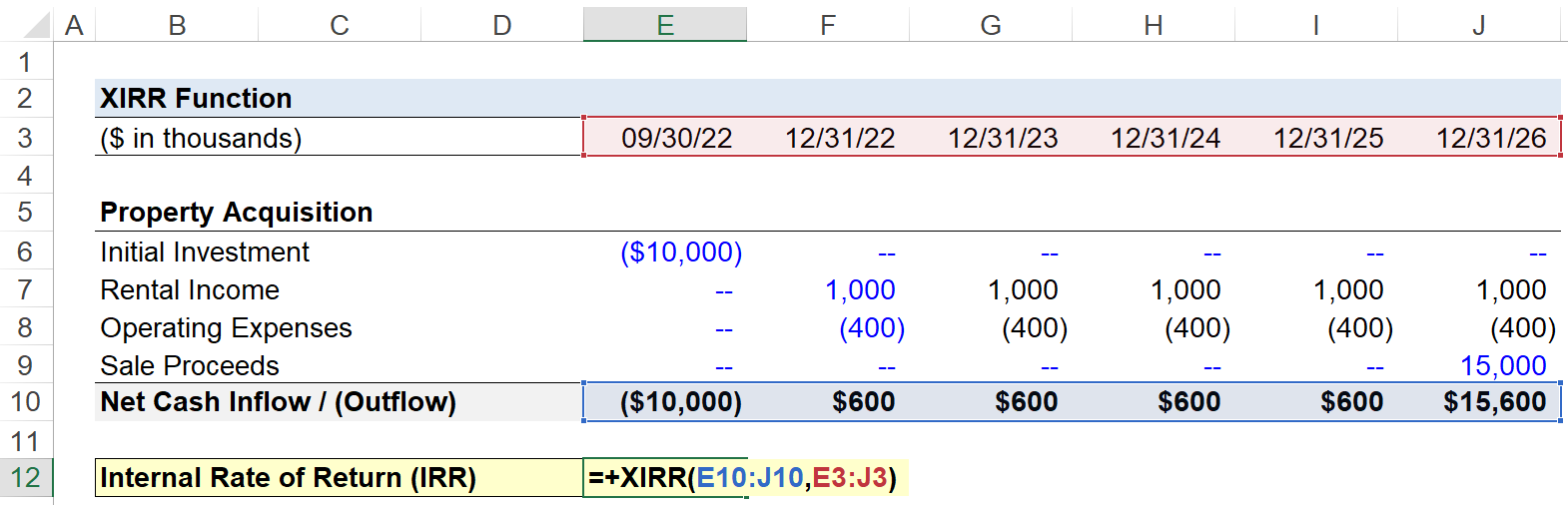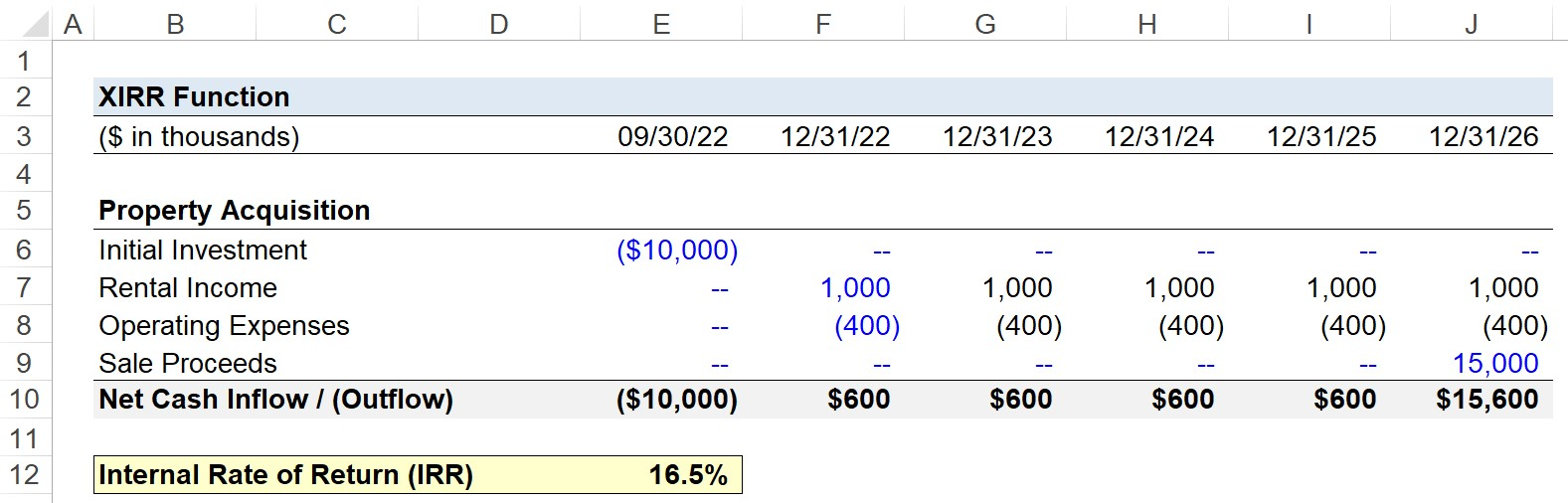# XIRR Function

Guide to Understanding the Excel "XIRR" Function## How to Use the XIRR Function in Excel (Step-by-Step)

The XIRR function in Excel computes the internal rate of return (IRR), which refers to the compounded rate of return on a specific investment.

In other words, the internal rate of return (IRR) is the interest rate that an initial investment must have grown by each year in order to reach the provided value at exit – i.e. from the beginning value to the ending value.

The XIRR function yields the implied internal rate of return (IRR) given a schedule of cash inflows and outflows.

But unique to the XIRR function, the cash flows are NOT necessarily required to be periodic, i.e. the dates at which the cash flows occur can be irregular with regard to timing.

The XIRR Excel function requires two inputs, which are the following:

1. The Range of Cash Inflows / (Outflows)
2. The Range of Dates Corresponding to Each Specific Cash Flow

## XIRR Function Formula

The XIRR function formula in Excel is as follows:

=XIRR(values, dates, [guess])

In order for the formula to work properly, you must enter the cash inflows and outflows directly in line with the corresponding dates – otherwise, the calculated IRR will be incorrect.

The range of cash values must also contain at least one positive and one negative number.

Under the context of investing, the initial investment should be entered as a negative figure since it represents an outflow of cash.

• Cash Outflows ➝ Negative Number
• Cash Inflows ➝ Positive Number

Inflows of cash could potentially include dividends received during the holding period and the sale proceeds on the date of exit.

## XIRR Excel Function Syntax

The table below describes the syntax of the Excel XIRR function in more detail.

Argument Description Required?
values
• The series of cash inflows and outflows that correspond to the dates stated in the schedule.
• Required
dates
• The specific dates that correspond to the payments in the “values” array.
• Required
guess
• An optional guess of the internal rates of return (IRR) — most often omitted (i.e. left blank).
• Optional

## XIRR vs. IRR Excel Function: What is the Difference?

The XIRR function in Excel is more practical than the IRR function due to the increased flexibility of not being restricted to annual periods.

Unlike the IRR function, XIRR can handle irregular cash flows, which reflects reality more accurately.

The drawback to the IRR function is that Excel assumes each cell is separated by exactly twelve months, which is rarely actually the case.

=IRR(values, [guess])

While the “IRR” Excel function can be used to calculate the return on a series of periodic, annual cash flows (i.e. evenly spaced out with one year in between), the “XIRR” function tends to be more practical on the job.

For XIRR, the effective annual rate is returned with daily compounding, while the IRR function assumes a stream of equally spaced, annual cash flows.

## XIRR Function Calculator – Excel Model Template

We’ll now move on to a modeling exercise, which you can access by filling out the form below.Submitting ...

## Step 1. Real Estate Acquisition Assumptions

Suppose a real estate investor purchased a property for \$10 million on 9/30/2022, with the intent to put it back on the market in approximately five years.

• Initial Investment = \$10 million
• Purchase Date = 09/30/22

After a couple of months of searching for tenants, the investor manages to earn \$1 million in rental income for the next five years.

With regard to the operating expenses incurred by the investor, we’ll assume there is \$400k in annual OpEx throughout the five-year time span, for the sake of simplicity.

12/31/22 to 12/31/26

• Annual Rental Income = \$1 million
• Annual Operating Expenses = (\$400,000)

At the end of fiscal year 2026, the investor is able to sell the property for \$15 million.

• Sale Proceeds = \$15 million

## Step 2. IRR Calculation Using Excel XIRR Function Example

Since our returns schedule is set up, we can calculate the internal rate of return (IRR) from the acquisition using the XIRR function in Excel.

But for each of the four items, it is necessary that the sign conventions were entered properly, or else the IRR calculation will be incorrect.

The initial investment and operating expenses represent “cash outflows” (–), whereas the rental income and sale proceeds reflect “cash inflows” (+).

Once we calculate the sum in the “Net Cash Inflow / (Outflow)” line item, the only remaining step is to use the XIRR function, where we’ll first select the array of net cash flows, followed by the corresponding dates.

=XIRR(E10:J10,E3:J3)The implied internal rate of return (IRR) earned from the property acquisition comes out as 16.5%.

If we had used the “IRR” Excel function instead, the calculated IRR is 13.6%, which is incorrect because it wrongly assumes that the initial quarter stub period is a full one-year period. The IRR is lower in comparison because the IRR yield declines with longer holding periods.

Hence, XIRR is the more practical Excel function to use when working with uneven cash flows, where the cash flows occur at irregular dates.Turbo-charge your time in Excel Used at top investment banks, Wall Street Prep's Excel Crash Course will turn you into an advanced Power User and set you apart from your peers.Inline Feedbacks';
Learn Excel Online

Become an Excel “Power User.” Used to train new hires at top financial institutions around the world!

X

The Wall Street Prep Quicklesson Series

7 Free Financial Modeling Lessons

Get instant access to video lessons taught by experienced investment bankers. Learn financial statement modeling, DCF, M&A, LBO, Comps and Excel shortcuts.